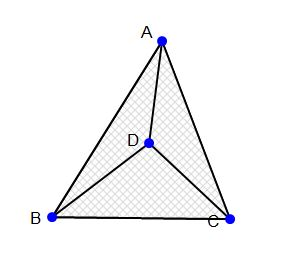# Center Centroid

Geometry Level 3$\frac {AD^2 + BD^2 + CD^2}{AB^2 + BC^2 + CA^2}$

The picture shows a $\Delta ABC$ with centroid $D$. What is the value of the expression above? Give your answer to three decimal places.

×